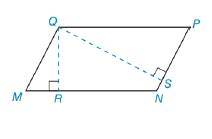Chapter 8.1, Problem 49E### Elementary Geometry for College St...

6th Edition
Daniel C. Alexander + 1 other
ISBN: 9781285195698

#### Solutions

Chapter
Section### Elementary Geometry for College St...

6th Edition
Daniel C. Alexander + 1 other
ISBN: 9781285195698
Textbook Problem
14 views

# In Exercises 47 to 50, use the fact that the area of the polygon is unique.In ▱ M N P Q ,   Q P = 12 and Q M = 9. The length of altitude Q R ¯ (to side M N ¯ ) is 6. Find the length of a altitude Q S ¯ from Q to P N ¯ .To determine

To find:

The length of the altitudes

Explanation

Converting theoretical statement into mathematical statement, identifying the geometric figure and the calculation is done by suitable geometric formulae.

Calculation:

Given,

Here, MN=b=12

QR=h=6

Area of the parallelogram A=bh unit2

=(12)(6)

=72 unit2

Find QS¯

For Q to PN¯

Choosing

### Still sussing out bartleby?

Check out a sample textbook solution.

See a sample solution

#### The Solution to Your Study Problems

Bartleby provides explanations to thousands of textbook problems written by our experts, many with advanced degrees!

Get Started

#### Find more solutions based on key concepts